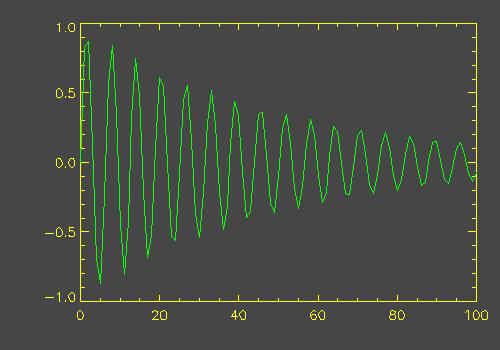# Graphics Without Graphics Windows

QUESTION: Is it possible to create a graphics plot and save it as a JPEG file without opening an IDL graphics window?ANSWER: Yes, it is possible. In fact, this is one indispensable function of the Z graphics buffer in IDL, which works completely in software without any interaction with hardware at all. The first thing you want to do is save your current graphics device and change to the Z graphics device, like this:

```   thisDevice = !D.Name
Set_Plot, 'Z', /COPY
```

The Copy keyword above is used to copy the current color table into the Z graphics buffer. You don't have to do this. You can load color tables once you are in the Z graphics buffer with exactly the same commands you use elsewhere in IDL.

The next step is to configure the Z graphics buffer. Typically, this means setting the "window" size and (for this operation) turning off the Z buffering capacity. You want the Z buffer to act more or less exactly like a graphics window. If Z buffering is turned on, the graphics window has "depth". This is how hidden line and surface removal is accomplished in the Z buffer. But as I say, for this operation, you want to turn it off. The Z device, like other graphics devices in IDL, is controlled by the Device command. Suppose we want a JPEG image of the plot 500 pixels wide by 350 pixels high. The Erase command is to erase anything that may have been left in the Z graphics buffer previously.

```   Device, Set_Resolution=[500,350], Z_Buffer=0
Erase
```

Now draw your graphics display.

```   colors = FSC_COLOR(['charcoal', 'yellow', 'green'], Indgen(3)+1)
data = Findgen(101)
data = Sin(data)/Exp(data/50)
Plot, data, Color=colors, Background=colors, /NoData
OPlot, data, Color=colors
```

At this point, you want to take a "snapshot" of the window's contents and you want to save the current color table vectors. You will need the color table vectors to create the 24-bit image you need to create the color JPEG file. The Z graphics buffer is an 8-bit device, so you cannot read a 24-bit image directly out of it like you could in a regular IDL graphics window on a 24-bit device. After you have taken the snapshot of the window's contents and saved the color table vectors, you are finished with the Z graphics buffer, so you can switch back to your normal graphics device. It might be a good idea to turn Z buffering back on, or you might forget it was off and wonder why the Z buffer didn't appear to be doing its job.

```   snapshot = TVRD()
TVLCT, r, g, b, /Get
Device, Z_Buffer=1
Set_Plot, thisDevice
```

To create a color JPEG file, you need a 24-bit image with the color information build into the image. We construct the 24-bit image like this.

```   image24 = BytArr(3, 500, 350)
image24[0,*,*] = r[snapshot]
image24[1,*,*] = g[snapshot]
image24[2,*,*] = b[snapshot]
```

Finally, we create the JPEG file. You see what it looks like in the illustration below the code.

```   Write_JPEG, 'lineplot.jpg', image24, True=1, Quality=75
```## The Z-Graphics Buffer as a 24-Bit Device

As of IDL 6.4, the Z-graphics buffer is no longer strictly an 8-bit device. It can also be set up as a 24-bit device, which makes creating a true-color image as easy as it is on the 24-bit displays most of us use now. Note, however, that you are going to have to be a lot more aware of which color decomposition mode you are using. (This is no different from using the TV command. See the long PDF article Working with Color for additional information.

To write a JPEG file using the Z-buffer as a 24-bit device, you might type these commands:

```   thisDevice = !D.Name
Set_Plot, 'Z'
Erase
Device, Set_Resolution=[500,350],Set_Pixel_Depth=24, Decomposed=1
data = Findgen(101)
data = Sin(data)/Exp(data/50)
Plot, data, Color=FSC_Color('yellow'), Background=FSC_Color('charcoal'), /NoData
OPlot, data, Color=FSC_Color('green')
snapshot = TVRD(True=1)
Set_Plot, thisDevice
Write_JPEG, 'lineplot.jpg', snapshot, True=1, Quality=75
```[Return to IDL Programming Tips]